# Kimberling, Clark

## Special M ★★

Author(s): Kimberling

Letdenote the golden ratio,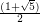and let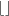denote the floor function. For fixed, let, let, and let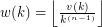. We can expectto have about the same growth rate as.

Conjecture   Prove or disprove that for every fixed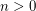, asranges through all the positive integers, there is a numbersuch that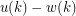takes each of the values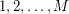infinitely many times, and. (Can you formulateas a function of? Generalize for other numbers?)

Keywords: